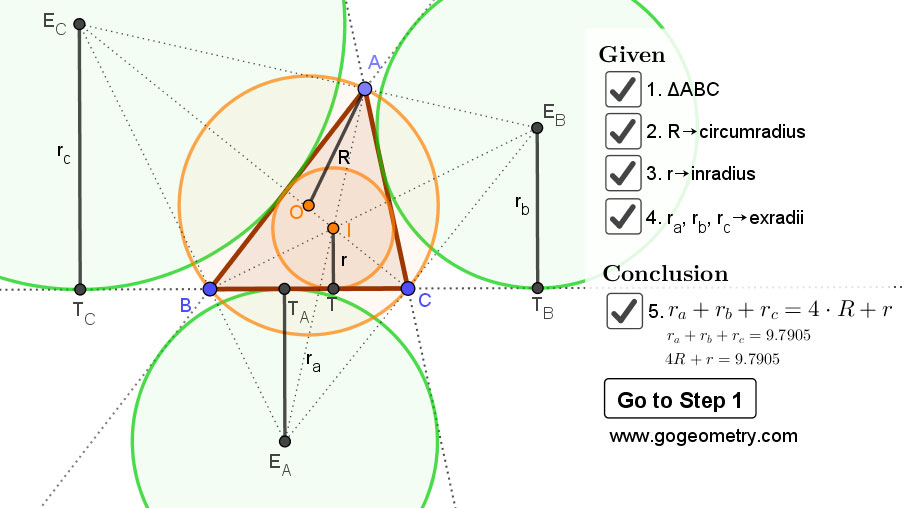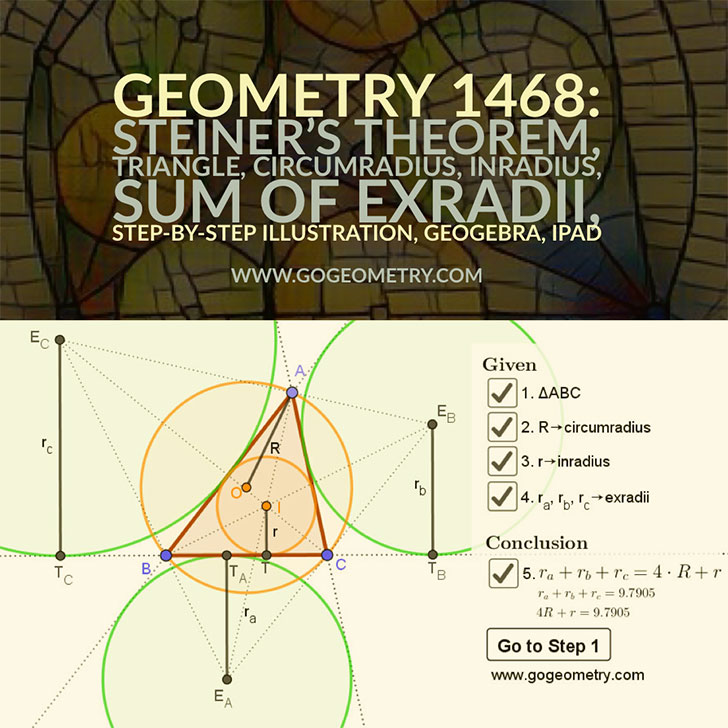# Dynamic Geometry 1468: Steiner's Theorem, Triangle, Circumradius, Inradius, Sum of Exradii, Step-by-step Illustration

The figure below shows a triangle ABC with the circumradius R, the inradius r and the exradii ra, rb, rc. Prove that $$r_a+r_b+r_c=4 \cdot R+r$$ .

## Static Diagram of Geometry 1468: Steiner's Theorem## Poster of the Dynamic Geometry 1468 using iPad Apps### Classroom Resource:Interactive step-by-step animation using GeoGebra

This step-by-step interactive illustration was created with GeoGebra.

• To explore (show / hide): click/tap a check box.
• To stop/play the animation: click/tap the icon in the lower left corner.
• To go to first step: click/tap the "Go to step 1" button.
• To manipulate the interactive figure: click/tap and drag the blue points or figures.

GeoGebra is free and multi-platform dynamic mathematics software for all levels of education that joins geometry, algebra, tables, graphing, statistics and calculus application, intended for teachers and students. Many parts of GeoGebra have been ported to HTML5.